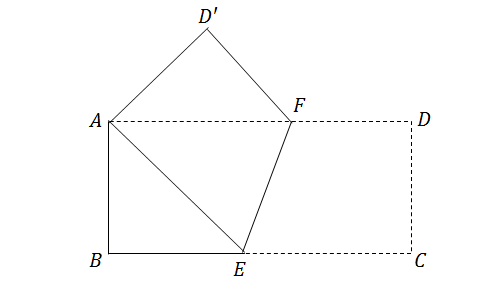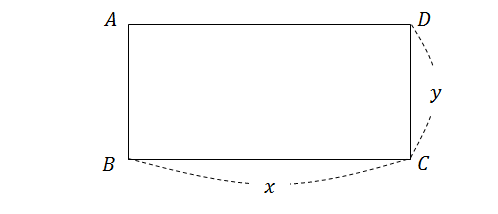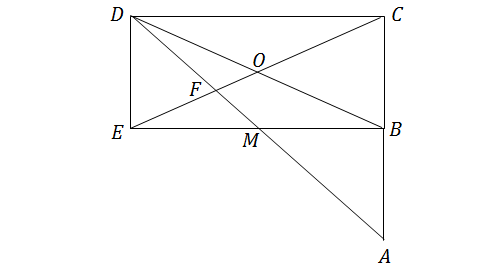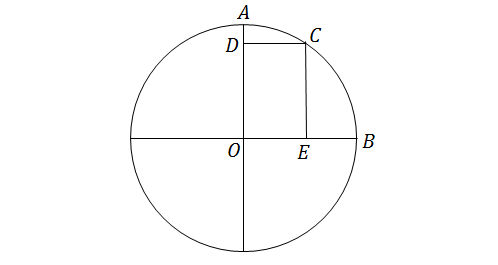Geometry

# Properties of RectanglesThe above diagram shows a rectangle creased at $EF$ such that that point $C$ aligns with point $A.$ If $\angle BAE=38^\circ,$ what is $\angle EFD$ in degrees?

Note: The above figure is not drawn to scale.The figure above depicts a rectangle with $\overline{AB}=5$ and $\overline{AD}=13.$ Find $x+y.$

$ABCD$ is a square. On line segment $AB$, $E$ is a point such that $\angle ADE = 37 ^\circ$. What is the measure of $\angle EDC$ (in degrees)?In the diagram above, $\square BCDE$ is a rectangle with horizontal side length $30$ cm and vertical side length $6$ cm. Line segments $\overline{BD}$ and $\overline{CE}$ are diagonals of $\square BCDE,$ which intersect at point $O$. Point $M$ is the bisector of $\overline{BE},$ and the extension of lines $\overline{DM}$ and $\overline{CB}$ intersect at point $A.$ If point $F$ is the intersection of $\overline{CE}$ and $\overline{AD},$ what is the area of $\triangle DOF?$

Note: The above figure is not drawn to scale.The diagram above shows a circle centered at $O,$ with rectangle $ODCE$ inscribed in the quarter circle $AOB.$ If the radius of the circle is $14$, what is the length of line segment $\overline{DE}?$

×